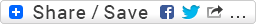## First steps with Canvas and HTML5

I’ve been postponing experimenting with HTML5 for quite a while, so today I finally set aside a few hours to play with it. This is a very simple example of the graphic effects you can create using javascript and the HTML5 canvas element..

Note that the examples below will work only on HTML5-compatible browsers, such as the latest versions of Chrome or Firefox..

### 1. Setting up a canvas and drawing some lines on it.

This is easily done using the moveTo and lineTo functions. So let’s create a simple symmetrical geometrical shape..

Your browser does not support the canvas element.

[inline]

[script type=”text/javascript”]

var c=document.getElementById(“myCanvas1”);
var cxt=c.getContext(“2d”);
var i=0;

for (i=1;i<=5;i++) { cxt.moveTo(0, 400); cxt.lineTo(100, i * 40); cxt.lineTo(300, i * 60); cxt.lineTo(400, i * 30); cxt.lineTo(500, i * 60); cxt.lineTo(700, i * 40); cxt.lineTo(800, 400); } cxt.stroke(); [/script] [/inline]

Here’s the source code:

```<!DOCTYPE HTML>
<html>
<body>

<canvas id="myCanvas1" width="800" height="400" style="border:1px dashed #c3c3c3;">
Your browser does not support the canvas element.
</canvas>

<script type="text/javascript">

var c=document.getElementById("myCanvas1");
var cxt=c.getContext("2d");
var i=0;

for (i=1;i<=5;i++)
{
cxt.moveTo(0, 400);
cxt.lineTo(100, i * 40);
cxt.lineTo(300, i * 60);
cxt.lineTo(400, i * 30);
cxt.lineTo(500, i * 60);
cxt.lineTo(700, i * 40);
cxt.lineTo(800, 400);
}

cxt.stroke();

</script>

</body>
</html>
```

### 2. Creating a mirroring effect

Now we can increase the size of the canvas to 800 and replicate these lines at the bottom of the canvas so to achieve a ‘mirroring’ effect.. since we know the max Y value of the canvas (800), let’s just add another loop that draws the same lines but inverts the Y position. This is easily achieved by subtracting the constant-dependent parameter from the max value of Y.

Your browser does not support the canvas element.

[inline]

[script type=”text/javascript”]

var c=document.getElementById(“myCanvas2”);
var cxt=c.getContext(“2d”);
var i=0;

for (i=1;i<=5;i++) { cxt.moveTo(0, 400); cxt.lineTo(100, i * 40); cxt.lineTo(300, i * 60); cxt.lineTo(400, i * 30); cxt.lineTo(500, i * 60); cxt.lineTo(700, i * 40); cxt.lineTo(800, 400); } for (i=5;i>=1;i–)
{
cxt.moveTo(0, 400);
cxt.lineTo(100, 800 – (i * 40));
cxt.lineTo(300, 800 – (i * 60));
cxt.lineTo(400, 800 – (i * 30));
cxt.lineTo(500, 800 – (i * 60));
cxt.lineTo(700, 800 – (i * 40));
cxt.lineTo(800, 400);
}

cxt.stroke();

[/script]

[/inline]

This is how the new source code looks like:

```<canvas id="myCanvas2" width="800" height="800" style="border:1px dashed #c3c3c3;">
Your browser does not support the canvas element.
</canvas>

<script type="text/javascript">

var c=document.getElementById("myCanvas2");
var cxt=c.getContext("2d");
var i=0;

for (i=1;i<=5;i++)
{
cxt.moveTo(0, 400);
cxt.lineTo(100, i * 40);
cxt.lineTo(300, i * 60);
cxt.lineTo(400, i * 30);
cxt.lineTo(500, i * 60);
cxt.lineTo(700, i * 40);
cxt.lineTo(800, 400);
}

for (i=5;i>=1;i--)
{
cxt.moveTo(0, 400);
cxt.lineTo(100, 800 - (i * 40));
cxt.lineTo(300, 800 - (i * 60));
cxt.lineTo(400, 800 - (i * 30));
cxt.lineTo(500, 800 - (i * 60));
cxt.lineTo(700, 800 - (i * 40));
cxt.lineTo(800, 400);
}

cxt.stroke();

</script>
```

### 3. Adding more graphical interest

Finally, let’s parametrize a bit more the construction of this geometrical pattern by including everything into another loop, and using this new counter to increment the points ordinate position. We’ll add a new variable y and use it to run the external loop 20 times. Here’s the result:

Your browser does not support the canvas element.

[inline]

[script type=”text/javascript”]

var c=document.getElementById(“myCanvas3”);
var cxt=c.getContext(“2d”);
var i=0;
var y=0;

for (y=1;y<=20;y++) { for (i=1;i<=5;i++) { cxt.moveTo(0, 400+y); cxt.lineTo(100, i * (40+y)); cxt.lineTo(300, i * (60+y)); cxt.lineTo(400, i * (30+y)); cxt.lineTo(500, i * (60+y)); cxt.lineTo(700, i * (40+y)); cxt.lineTo(800, 400+y); } for (i=5;i>=1;i–)
{
cxt.moveTo(0, 400);
cxt.lineTo(100, 800 – (i * (40+y)));
cxt.lineTo(300, 800 – (i * (60+y)));
cxt.lineTo(400, 800 – (i * (30+y)));
cxt.lineTo(500, 800 – (i * (60+y)));
cxt.lineTo(700, 800 – (i * (40+y)));
cxt.lineTo(800, 400+y);
}

}

cxt.stroke();

[/script]

[/inline]

Not too bad uh? This last modification to the code looks like this:

```<canvas id="myCanvas3" width="800" height="800" style="border:1px dashed #c3c3c3;">
Your browser does not support the canvas element.
</canvas>

<script type="text/javascript">

var c=document.getElementById("myCanvas3");
var cxt=c.getContext("2d");
var i=0;
var y=0;

for (y=1;y<=20;y++)
{

for (i=1;i<=5;i++)
{
cxt.moveTo(0, 400+y);
cxt.lineTo(100, i * (40+y));
cxt.lineTo(300, i * (60+y));
cxt.lineTo(400, i * (30+y));
cxt.lineTo(500, i * (60+y));
cxt.lineTo(700, i * (40+y));
cxt.lineTo(800, 400+y);
}

for (i=5;i>=1;i--)
{
cxt.moveTo(0, 400);
cxt.lineTo(100, 800 - (i * (40+y)));
cxt.lineTo(300, 800 - (i * (60+y)));
cxt.lineTo(400, 800 - (i * (30+y)));
cxt.lineTo(500, 800 - (i * (60+y)));
cxt.lineTo(700, 800 - (i * (40+y)));
cxt.lineTo(800, 400+y);
}

}

cxt.stroke();

</script>
```

### Cool! I’d like to know more..

Yes, I agree. This opens up a new world for web-based graphics.. and I just scratched the surface of it! In particular I’d like to see how this type of graphical components can be used to compose visualizations in the digital humanities – provided more interactivity is added to them.

Here are a couple if learning resources I found useful:

• Learning the basics of HTML5 canvas on .Net magazine online
• Canvas tutorial on Mozilla.org (more advanced)
• Javascript Graphics and Effects Frameworks: useful document providing a list of the most common libraries for doing javascript-based graphics
• Canvas and interactivity tutorial: a step by step discussion on how to create a ‘breakout’ game clone that you can play in your browser, using javascript and the canvas element
• Creating an HTML 5 canvas painting application: nice tutorial that shows how to put together several canvas drawing techniques so to build a basic ‘painting’ application
• 21 Ridiculously Impressive HTML5 Canvas Experiments: a collection of some state-of-the-art HTML5 canvas-based experiments that will make you say, “Wow!”
•